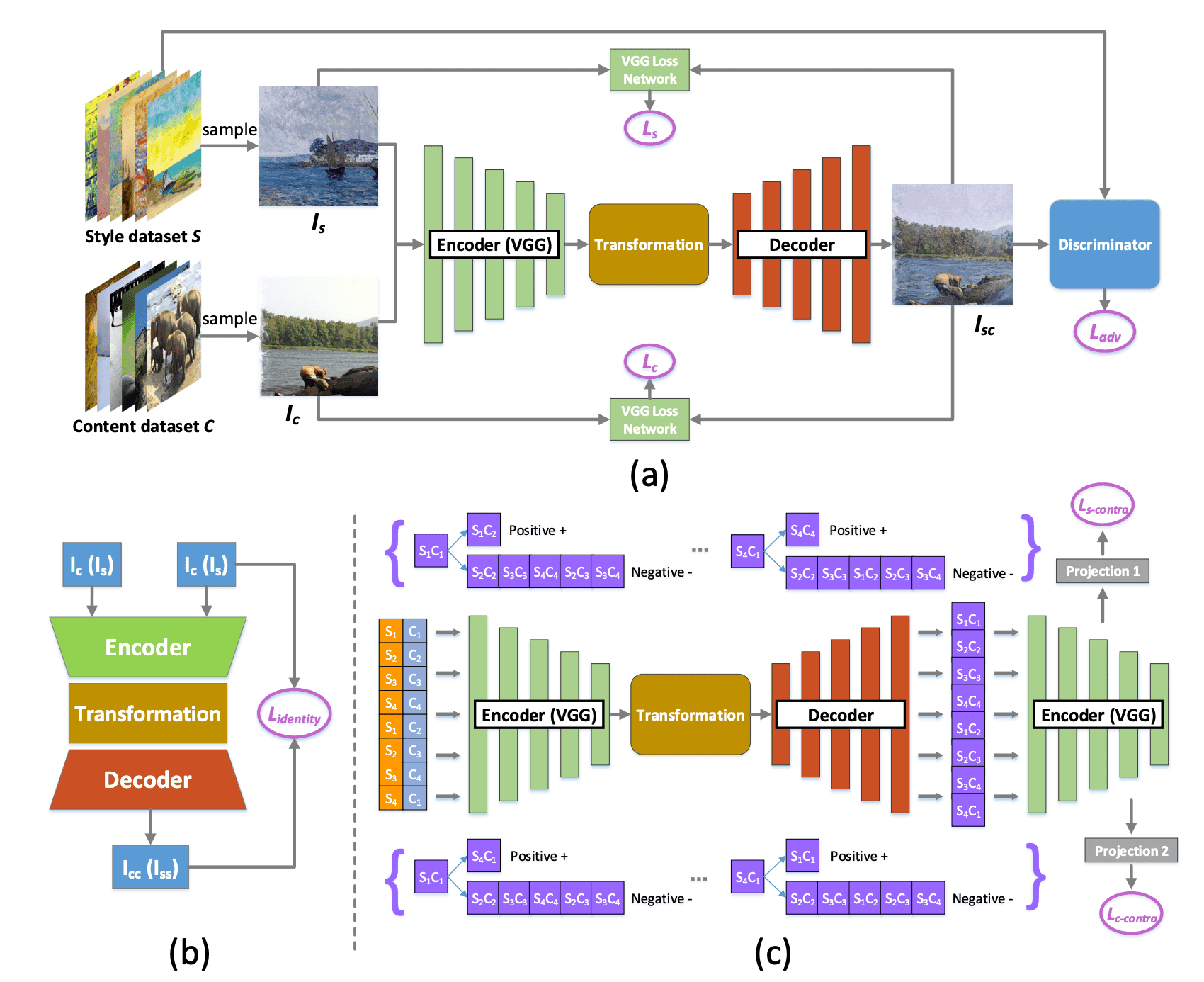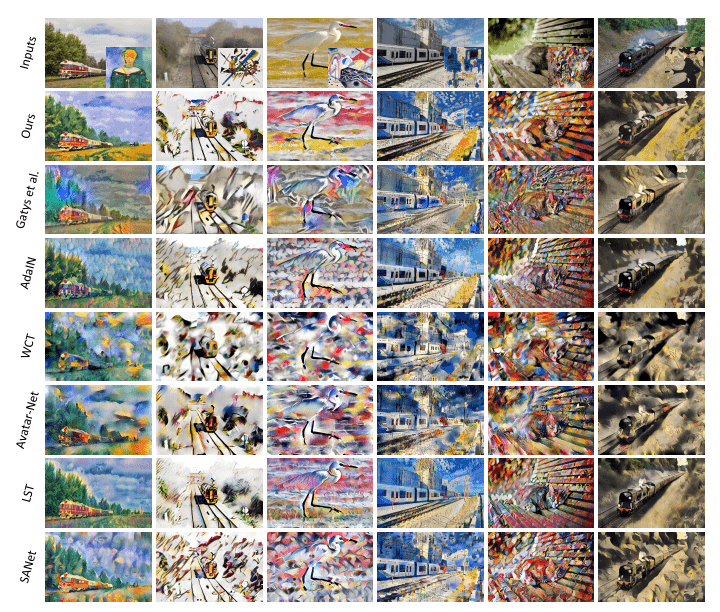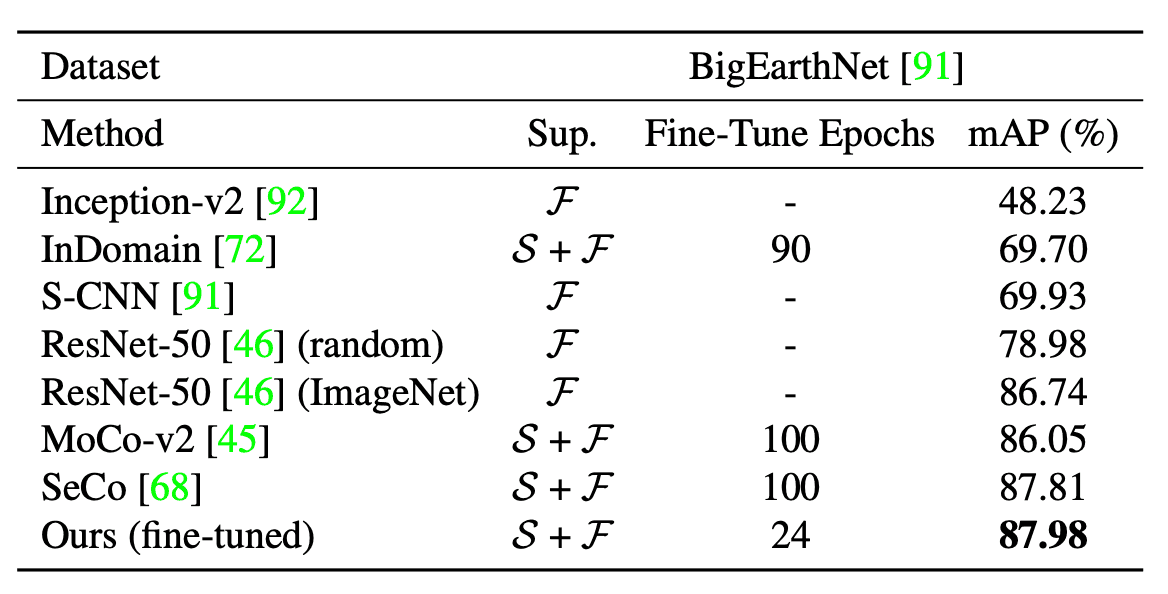# Catch up on the latest AI articles# Art Style Transformation Using Internal-external Learning And Contrastive Learning

3 main points
✔️ A new internal-external style transformation that takes into account internal and external learning significantly bridges the gap between human-generated and AI-generated pictures.
✔️ For the first time, we introduced Contrastive learning into style transformation to obtain more complete style transformation results with learned relationships between styles.
✔️ Compared its effectiveness and superiority with several existing SOTA methods.

written by Haibo ChenLei ZhaoZhizhong WangZhang Hui MingZhiwen ZuoAilin LiWei XingDongming Lu
(Submitted on 22 May 2021)

Subjects: Computer Vision and Pattern Recognition (cs.CV)code：The images used in this article are from the paper, the introductory slides, or were created based on them.

## first of all

Existing art style transformations produce excellent results with deep neural networks, but they produce discordant colors and repeated patterns. Therefore, in this paper, we proposed internal-external learning with two contrastive losses. In particular, we used the internal statistics of a single style image to determine the color and texture and extracted the external information from a large-scale style dataset to make the color and pattern more harmonious. Furthermore, noting that existing models consider content-stylization and style-stylization relationships, but not stylization-stylization relationships, for the inclusion of multiple stylized images, we introduced a Contrastive loss that makes those that share content or style close and those that do not share them distant We introduced a Contrastive loss that makes the

## technique

The schematic diagram of our method is shown in the figure below. For the backbone, we used SANet, one of the SOTA models of style transformation.### Internal-external learning

Let $C$ denote the dataset of photos and $S$ the art dataset. Our goal is to learn both the internal style features from a single art $I_S\in S$ and the human-aware external style features from $S$ to obtain a style-transformed artistic image $I_{SC}$ of any content $I_C\in C$.

### Internal style learning

Based on existing methods, we used the trained VGG-19 model $\phi$ to obtain the internal style features of a single art image. The style loss is as follows.

$${\cal L}_S:=\sum_{i=1}^{L}||\mu(\phi_i(I_{SC}))-\mu(\phi_i(I_S))||_2+|\sigma(\phi_i(I_{SC}))-\sigma(\phi_i(I_S))||_2$$

where $\phi_i$ denotes the $i$th layer of $\phi$. $\mu,\sigma$ are mean and variance, respectively.

### external style learning

To learn human-aware styles from $S$, we use GAN, where the Generator is ${\cal G}$ and the Discriminator is ${\cal D}$, the false images are stylized images and the true images are artistic images. The adversarial loss is as follows.

$${\cal L}_{adv}:={\mathbb E}_{I_S\sim S}[log({\cal D}(I_S))+{\cal E}_{I_C\sim C, I_S\sim S}log(1-{\cal D}(D(T(E(I_C), E(I_S)))))]$$

### Content structure preservation

To keep the structure of the content image, we introduce the following loss

$${\cal L}_C:=||\phi_{conv4\_2}(I_{SC})-\phi_{conv4\_2}(I_C)||_2$$

### Identity loss

If the content and style images are identical, then Generator ${\cal G}$ should map them identically. This preserves content structure and style structure. identity loss is computed as follows

$${\cal L}_{identity}:=\lambda_{identity1}(||I_{CC}-I_C||_2+|||I_{SS}-I_S|||2)+\lambda_{identity2}\sum_{i=1}^L(||\phi_i(I_{CC})-\phi_i(I_C)||_2+||\phi_i(I_{SS})-\phi_i(I_S)||_2)$$

where $I_{CC}$ is the generated image when the content image and style image are $I_C$, and $I_{SS}$ is the same. $\lambda_{identity}$ is the balance parameter.

### Contrastive learning

Intuitively, styled transformed images with the same styled image should have a close relationship, and styled transformed images with the same content should also have a close relationship. These relationships are called stylization-stylization relationships. Conventional methods do not consider these relations, such as ${\cal L}_S$ and ${\cal L}_C$. such as ${Contrastive L}_S$ and ${Cal L}_C$. Therefore, in this paper, we introduce Contrastive learning and consider these relations. In particular, we define two types of Contrastive loss for style and content. Let $s_i, c_i$ be the $i$-th style image and content image, respectively, and $s_ic_i$ be the $c_i$. the image transformed by $s_i$'s style. Let $b$ (even) be the batch size, $\{s_1,s_2,\cdots,s_{b/2},s_1,s_2,\cdots,s_{b/2-1},s_{b/2}\}$ for the style batch, $\{c_1,c_2,\cdots,c_{b/2},c_2,c_3 ,\cdots,c_{b/2},c_1\}$. By doing so, for any $s_ic_j$, we can find $s_ic_x(x\neq j)$ that shares style and $s_yc_j(y\neq i)$ that shares content.

### Style contrastive loss

For a stylized image $s_ic_j$, with $s_ic_x(x\neq j)$ as a positive sample and $s_mc_n(m\neq i, n\neq j)$ as a negative sample, the style contrastive loss is as follows.

$${\cal L}_{S-contra}:=-log(\frac{exp(l_S(s_ic_j)^Tl_S(s_ic_x)/\tau)}{exp(l_S(s_ic_j)^Tl_S(s_ic_x)/\tau)+\sum exp(l_S(s_ic_j)^Tl_S(s_mc_n)/\tau)})$$

However, $l_S=h_S(\phi_{relu3\_1}(\cdot)), h_S$ is the style mapping network and $\tau$ is the temperature parameter.

### Content contrastive loss

Similarly, for $s_ic_j$, if $s_yc_j(y\neq i)$ is a positive sample and $s_mc_n(m\neq i, n\neq j)$ is a negative sample, the content contrastive loss is as follows.

$${\cal L}_{C-contra}:=-log(\frac{exp(l_C(s_ic_j)^Tl_C(s_yc_j)/\tau)}{exp(l_C(s_ic_j)^Tl_C(s_yc_j)/\tau)+\sum exp(l_C(s_ic_j)^Tl_C(s_mc_n)/\tau)})$$

However $l_C=h_C(\phi_{relu4\_1}(\cdot)), h_C$ is the content mapping network.

### Final Loss

The final loss function summarizes the above and returns

$${\cal L}_{final}:=\lambda_1{\cal L}_S+\lambda_2{\cal L}_{adv}+\lambda_3{\cal L}_C+\lambda_4{\cal L}_{identity}+\lambda_5{\cal L}_{S-contra}+\lambda_6{\cal L_{C-contra}}$$

The $GetHealth() method is used to create a new hyperparameter. where$\lambda\$ is a hyperparameter.

## result

### qualitative evaluation

The result is shown in the figure below: the first line shows the content image and style image, and the second and subsequent lines show the result of the style transformation using each method.

From the figure, we can see that the existing method shows distortion and shape collapse, whereas our method shows a harmonized style and plausible result.### quantitative evaluation

As a quantitative evaluation, we used the widely used LPIPS. To measure stability and agreement, we calculated the average distance between adjacent frames of the video. The results are shown in the table below. The lower the value, the better the performance, and our method gave the best results.## summary

This paper proposes an internal-external style transformation method with two contrastive losses. The results of various experiments show that our method outperforms existing methods both qualitatively and quantitatively. Since our method is simple and efficient, it gives a new understanding to the study of art style transformation and we aim to apply it to other methods in the future.

If you have any suggestions for improvement of the content of the article,